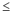# Example of getting and interpreting a p-value

You want to determine whether a new gasoline additive has an effect on gas mileage. If the known gas mileage for this specific class of cars is 25 miles per gallon (mpg), the hypotheses for this study are H0: μ = 25 and HA: μ ≠ 25.

## Getting the p-value from a 1-sample t-test

You test 35 cars and discover that the miles per gallon the cars get ranges from 14.4 to 28.8. After putting the data in the column MPG, you perform Minitab’s t-test (the menu command Stat > Basic Statistics > 1-Sample t, or the session command TTEST) and get these results:

### One-Sample T: C1

Descriptive Statistics N Mean StDev SE Mean 95% CI for μ 35 23.657 3.416 0.577 (22.484, 24.831) μ: mean of C1
Test Null hypothesis H₀: μ = 25 Alternative hypothesis H₁: μ ≠ 25

## Interpreting the p-value

The results show that the mean of the 35-car sample is 23.657. But the mean miles per gallon of all cars of this type (μ) might still be 25. You need to know whether there is enough sample evidence to reject H0. The most common way is to compare the p-value to the significance level, α (alpha). α is the probability of rejecting H0 when H0 is true. In this case, that’s the probability of concluding that the population mean is not 25 mpg, when in fact it is.

The p-value is a measure of the strength of the evidence in your data against H0. Usually, the smaller the p-value, the stronger the sample evidence is for rejecting H0. More specifically, the p-value is the smallest value of α that results in the rejection of H0. For any value of p-value > α, you fail to reject H0, and for any value of p-valueα, you reject H0.

In our t-test example, the test statistic is a function of the mean, and the p-value is .026. This indicates that 2.6% of the samples of size 35, drawn from the population where μ = 25, will produce a mean that provides as strong (or stronger) evidence as the current sample that μ is not equal to 25. Ask yourself which is more likely: that μ = 25 and you just happened to select a very unusual sample; or that μ is not equal to 25?

The p-value is traditionally compared to α values of less than .05 or .01, depending on the field of study. Check journal topics in your field for acceptable values.

In our example, let’s assume a .05 value of α. The p-value of .026 indicates that the mean miles per gallon of all cars of this type (not only the mean of the 35 cars in the study) is probably not equal to 25. A more statistically correct way to state this is “at a significance level of .05, the mean miles per gallon seems to be significantly different from 25.”

Using p-values, then, is simple if you know two key facts: the values of α that are acceptable in your field, and the null and alternative hypotheses for the tests you are using.

By using this site you agree to the use of cookies for analytics and personalized content.  Read our policy